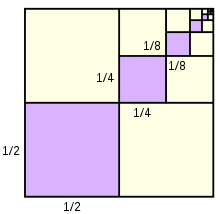# Quantities 60183

Determine the remaining quantities in the finite geometric sequence, given: n = 4, an = 12.5, sn = 187.5, a1 = ?, q =?

a1 =  100
q =  0.5

### Step-by-step explanation:Did you find an error or inaccuracy? Feel free to write us. Thank you!

Tips for related online calculators
Do you have a linear equation or system of equations and looking for its solution? Or do you have a quadratic equation?